# 6 Environments

When you use the Latex shortcode in Pressbooks, you start in math mode. When writing Latex outside of WordPress, you need to put ‘$’s around your equation to use math mode. It is helpful to remember this if you are looking at other tutorials or examples online, as their code might not work if you copy and paste it into Pressbooks without removing the ‘$’s.

Latex supports a variety of environments to create equations, tables, figures, and more. Equations are particularly helpful in Pressbooks, as they keep track of numbering.

For example, we can typeset the double-angle trigonometric identities as:

(1)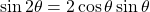(2)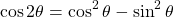[‌latex] \begin{equation} \sin 2 \theta = 2 \cos \theta \sin \theta \end{equation} [/latex] [‌latex] \begin{equation} \cos 2 \theta = \cos^2 \theta - \sin^2 \theta \end{equation} [/latex]

If you type text in math mode, the spacing will look odd as Latex will try to interpret the words as an equation. For example: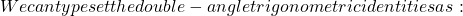[‌latex]We can typeset the double-angle trigonometric identities as:[/latex]

You can have Latex format text normally in math mode using the command \text{}.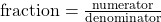[‌latex]\text{fraction} = \frac{\text{numerator}}{\text{denominator}}[/latex]

Latex also distinguishes between a math mode for typesetting text inline and a display format for stand-alone equations. In the solutions to the practice for commands, we saw that using \displaystyle switched Latex to using the mode for display, which looks nicer for large elements. Using the stand-alone mode will also make our fraction look nicer.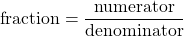[‌latex]\displaystyle \text{fraction} = \frac{\text{numerator}}{\text{denominator}}[/latex]

In addition to \displaystyle and the equation environment, you will often see people surround an equation with ‘’ to begin and end a display environment.

You don’t need to use many of the other Latex environments in Pressbooks, as tables and figures can be added using the WYSIWYG editor. However, if you decide to write stand-alone Latex documents you will use other environments frequently.